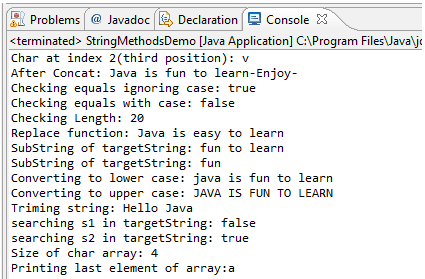# Exploring Methods of String Class

## Introduction

String manipulation is arguably one of the most common activities in computer programming. String class has a variety of methods for string manipulation. We will discuss basic methods with examples.

public char charAt(int index)

This method requires an integer argument that indicates the position of the character that the method returns.This method returns the character located at the String's specified index. Remember, String indexes are zero-based—for example,

```String x = "airplane";
System.out.println( x.charAt(2) ); // output is 'r'
```

public String concat(String s)

This method returns a String with the value of the String passed in to the method appended to the end of the String used to invoke the method—for example,

```String x = "book";
System.out.println( x.concat(" author") ); // output is "book author"
```

The overloaded + and += operators perform functions similar to the concat()method—for example,

```String x = "library";
System.out.println( x + " card"); // output is "library card"
String x = "United";
x += " States"
System.out.println( x ); // output is "United States"
```

public boolean equalsIgnoreCase(String s)

This method returns a boolean value (true or false) depending on whether the value of the String in the argument is the same as the value of the String used to invoke the method. This method will return true even when characters in the String objects being compared have differing cases—for example,

```String x = "Exit";
System.out.println( x.equalsIgnoreCase("EXIT")); // is "true"
System.out.println( x.equalsIgnoreCase("tixe")); // is "false"
```

public int length()

This method returns the length of the String used to invoke the method—for example,

```String x = "01234567";
System.out.println( x.length() ); // returns "8"
```

public String replace(char old, char new)

This method returns a String whose value is that of the String used to invoke the method, updated so that any occurrence of the char in the first argument is replaced by the char in the second argument—for example,

```String x = "oxoxoxox";
System.out.println( x.replace('x', 'X') ); // output is  "oXoXoXoX"
```

public String substring(int begin)/ public String substring(int begin, int end)

The substring() method is used to return a part (or substring) of the String used to invoke the method. The first argument represents the starting location (zero-based) of the substring. If the call has only one argument, the substring returned will include the characters to the end of the original String. If the call has two arguments, the substring returned will end with the character located in the nth position of the original String where n is the second argument. Unfortunately, the ending argument is not zero-based, so if the second argument is 7, the last character in the returned String will be in the original String's 7 position, which is index 6. Let's look at some examples:

```String x = "0123456789"; // the value of each char is the same as its index!
System.out.println( x.substring(5) ); // output is "56789"
System.out.println( x.substring(5, 8)); // output is "567"
```

public String toLowerCase()

This method returns a String whose value is the String used to invoke the method, but with any uppercase characters converted to lowercase—for example,

```String x = "A New Java Book";
System.out.println( x.toLowerCase() ); // output is "a new java book"
```

public String toUpperCase()

This method returns a String whose value is the String used to invoke the method, but with any lowercase characters converted touppercase—for example,

```String x = "A New Java Book";
System.out.println( x.toUpperCase() ); // output is"A NEW JAVA BOOK"
```

public String trim()

This method returns a String whose value is the String used to invoke the method, but with any leading or trailing blank spaces removed—for example,

```String x = " hi ";
System.out.println( x + "x" ); // result is" hi x"
System.out.println(x.trim() + "x"); // result is "hix"
```

public char[ ] toCharArray( )

This method will produce an array of characters from characters of String object. For example

```String s = “Java”;
Char [] arrayChar = s.toCharArray();  //this will produce array of size 4
```

public boolean contains(“searchString”)

This method returns true of target String is containing search String provided in the argument. For example-

```String x = “Java is programming language”;
System.out.println(x.contains(“Amit”)); // This will print false
System.out.println(x.contains(“Java”)); // This will print true
```

Below program demonstrate all above methods.

Java Code: Go to the editor

``````public class StringMethodsDemo {
public static void main(String[] args) {
String targetString = "Java is fun to learn";
String s1= "JAVA";
String s2= "Java";
String s3 = "  Hello Java  ";

System.out.println("Char at index 2(third position): " + targetString.charAt(2));
System.out.println("After Concat: "+ targetString.concat("-Enjoy-"));
System.out.println("Checking equals ignoring case: " +s2.equalsIgnoreCase(s1));
System.out.println("Checking equals with case: " +s2.equals(s1));
System.out.println("Checking Length: "+ targetString.length());
System.out.println("Replace function: "+ targetString.replace("fun", "easy"));
System.out.println("SubString of targetString: "+ targetString.substring(8));
System.out.println("SubString of targetString: "+ targetString.substring(8, 12));
System.out.println("Converting to lower case: "+ targetString.toLowerCase());
System.out.println("Converting to upper case: "+ targetString.toUpperCase());
System.out.println("Triming string: " + s3.trim());
System.out.println("searching s1 in targetString: " + targetString.contains(s1));
System.out.println("searching s2 in targetString: " + targetString.contains(s2));

char [] charArray = s2.toCharArray();
System.out.println("Size of char array: " + charArray.length);
System.out.println("Printing last element of array: " + charArray);

}

}
```
```

Output:Summary

• String manipulation is one of the most widely performed activities in java programming
• Java library is having various built-in methods like substring, concat, replace, converting to uppercase or lowercase etc

Java Code Editor:

Previous: String Class
Next:String buffer class and string builder class

﻿